# 自然语言处理 07：NLP 深度学习：前馈网络

## 墨尔本大学 COMP90042 课程笔记

Posted by YEY on March 25, 2020

# Lecture 07 NLP 深度学习：前馈网络

• 机器学习的一个分支。
• 它是神经网络的另一个名字。
• 神经网络：灵感来源于大脑的工作机制，包含被称为 神经元 的计算单元。
• 为什么是 深度？因为在现代深度学习模型中，有很多连接在一起的层。

## 1. 前馈神经网络

### 1.1 总体结构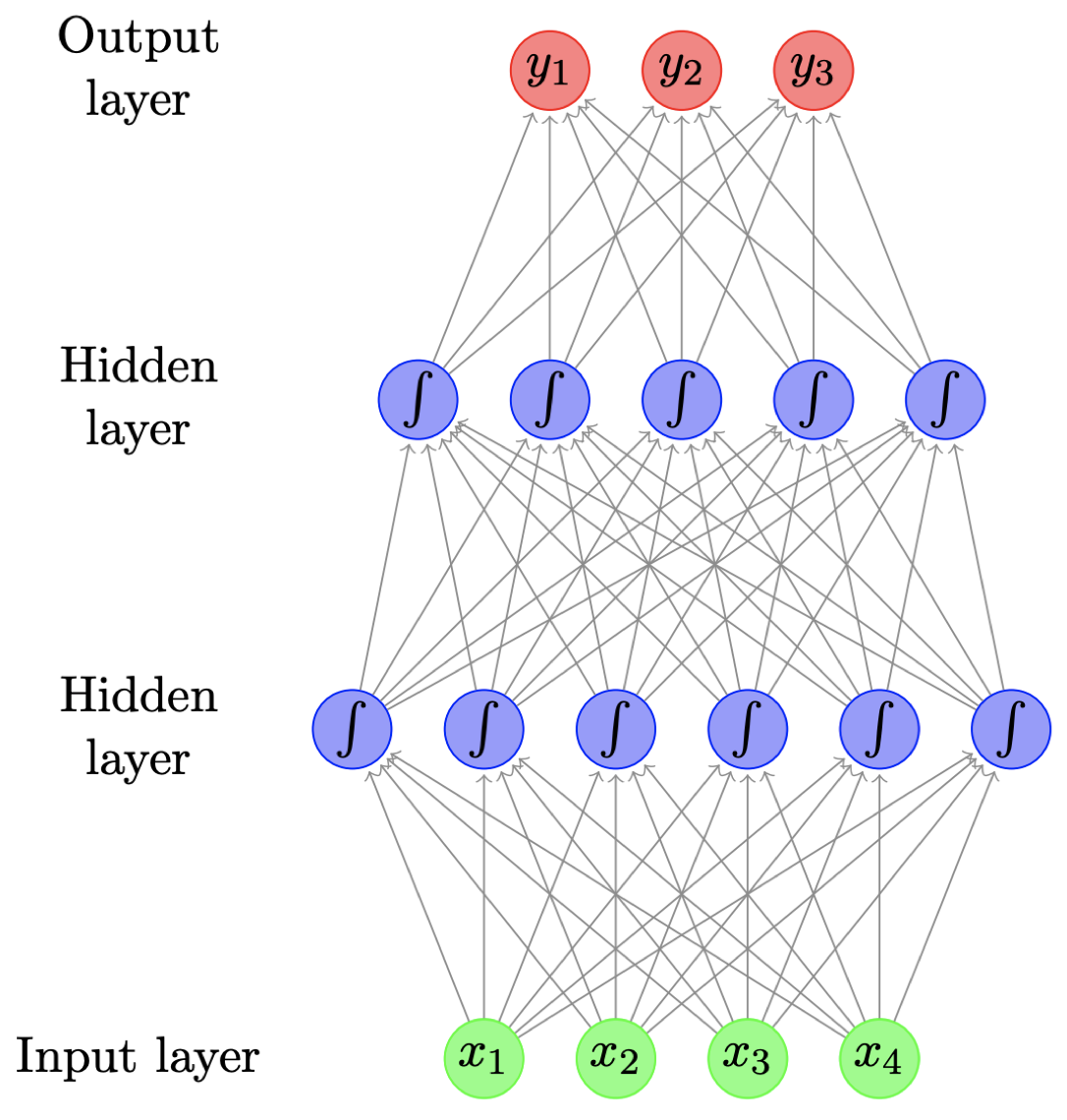• 又称 多层感知器（multilayer perceptrons）
• 上图是一个包含两个隐藏层的前馈神经网络，图中的每个圆圈表示一个神经元，它实际上对应着某个函数，该函数通常是一个非线性函数（例如：Sigmoid 函数），其输入来自前一层的输出的线性加权组合，而每个神经元的输出的线性组合将作为下一层的输入。以此类推，直到输出层，输出层通常是根据实际问题定制的，例如：处理一个三分类问题时，通常可以将输出层神经元个数设为 3 个。
• 图中的每个箭头都携带一个权重，其大小取决于输入变量的重要程度。
• Sigmoid 函数在这里引入了非线性。

### 1.2 神经元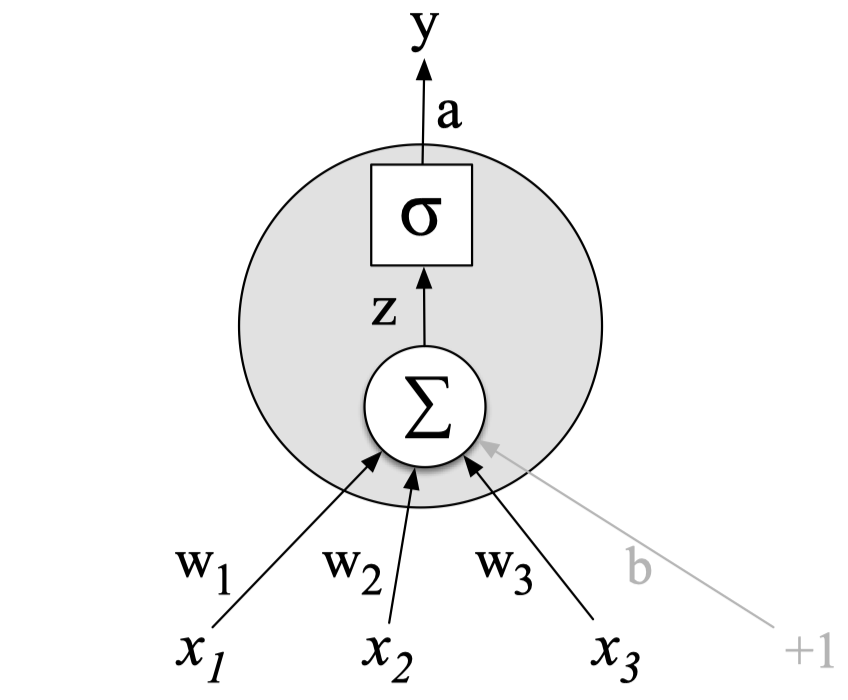• 给定输入（向量） $\mathbf x$，计算实值（标量）$h$

$h=\tanh \left(\sum_{j}w_jx_j+b\right)$
• 对输入进行缩放（乘以权重，$\mathbf w$），并且加上偏移量（偏置，$b$）
• 采用 非线性函数，例如：logistic sigmoid, hyperbolic sigmoid（tanh），或者 ReLU

### 1.3 矩阵向量表示

• 通常会有很多个隐藏神经元，即

$h_i=\tanh \left(\sum_{j}w_{ij}x_{j}+b_i\right)$
• 每个神经元都有属于自己的权重向量 $\mathbf w_i$ 和偏置项 $b_i$
• 某一层的计算结果可以用矩阵和向量操作表示

$\mathbf h=\tanh (W\mathbf x + \mathbf b)$

其中，$W$ 是一个由权重向量 $\mathbf w_i$ 构成的矩阵，$\mathbf x$ 是输入向量，$\mathbf b$ 是所有偏置项组成的向量。

• 此时，非线性函数实际上作用在每个元素上。

### 1.4 输出层

• 二分类问题（例如：对一条推文进行正面/负面情感分类）
• 使用 Sigmoid 激活函数（又称 Logistic 函数）
• 多分类问题（例如：对一个文档的主题进行分类）
• 使用 Softmax 函数，确保概率大于 $0$，并且和为 $1$。

$\left[\dfrac{\exp(v_1)}{\sum_i\exp(v_i)},\dfrac{\exp(v_2)}{\sum_i\exp(v_i)},\dots,\dfrac{\exp(v_m)}{\sum_i\exp(v_i)} \right]$

### 1.5 前馈神经网络$\mathbf h_1=\tanh (W_1\mathbf x + \mathbf b_1)$ $\mathbf h_2=\tanh (W_2\mathbf h_1 + \mathbf b_2)$ $\mathbf y=\text{softmax}(W_3\mathbf h_2)$

### 1.6 从数据中学习

• 本质上，模型试图尽可能地 “拟合” 训练数据，我们可以通过其分配给正确输出的概率来衡量：

$L=\prod_{i=0}^{m}P(y_i\mid x_i)$
• 最大化 所有训练数据的总概率 $L$
• 等价于 最小化 $\log L$，对于参数而言
• 训练采用梯度下降

• 很多工具，例如 tensorflow、pytorch、dynet 等，利用自动微分来自动计算梯度。

## 2. 应用

### 2.1 主题分类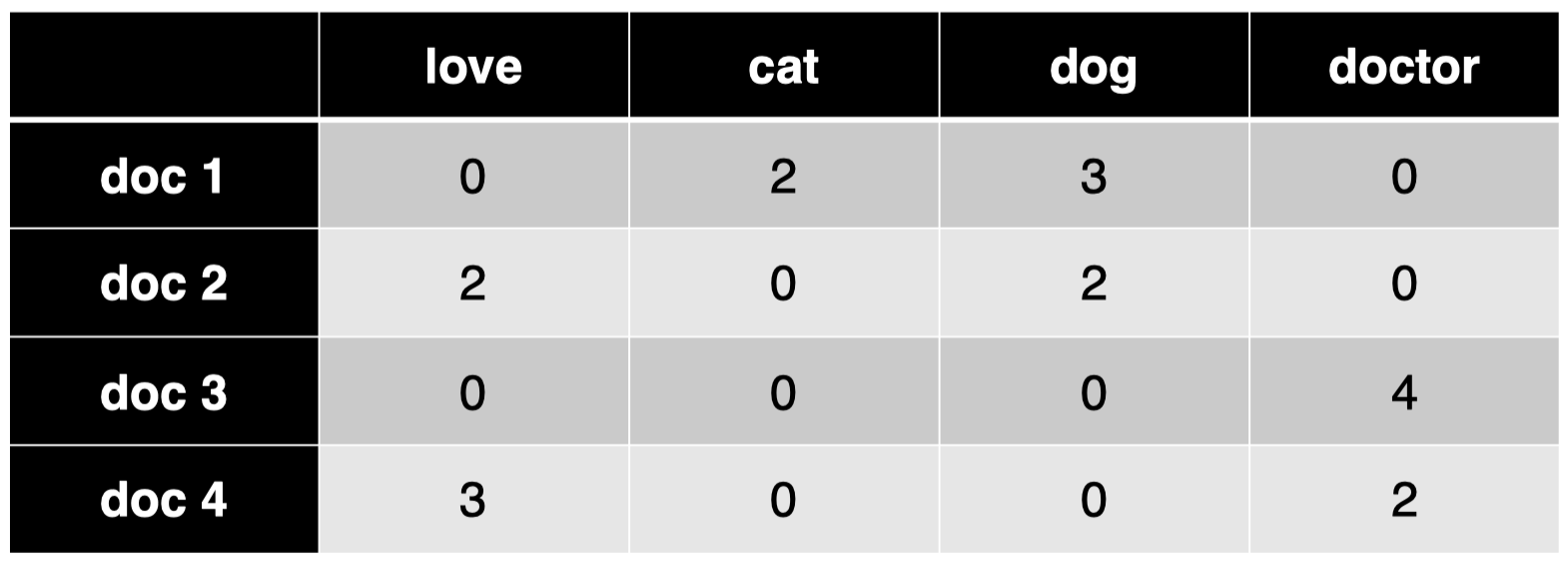$\mathbf h_1=\tanh (W_1\mathbf x + \mathbf b_1)$ $\mathbf h_2=\tanh (W_2\mathbf h_1 + \mathbf b_2)$ $\mathbf y=\text{softmax}(W_3\mathbf h_2)$

• 使用 bag-of-ngrams 作为输入。
• 文本预处理：词形还原、移除停用词等。
• 相比原始计数，我们可以用 TF-IDF 或者指示器（$0$ 或 $1$，取决于该单词是否存在）来进行单词加权。

### 2.2 作者身份识别

• 在该任务中，文本的风格特征要比单词内容本身更重要。
• POS tags 和功能词（例如：$\textit{on, of, the, and}$）
• 关于功能词，我们可以从特定的词典获得（因为功能词通常是一个封闭集合）。或者，另一种关于功能词很好的近似方法是：挑选一个大的语料库中出现频率最高的 300 个单词。

• 输入：bag of function words, bag of POS tags, bag of POS bigrams/trigrams
• 单词权重：密度（例如：在一个文本窗口内，功能词和内容词之间的数量比例）
• 其他特征：连续功能词之间距离的分布

## 3. 前馈神经网络语言模型

### 3.1 语言模型回顾

• 目标：为一个单词序列分配概率。
• 基本框架：可以视为句子上的 “滑动窗口”，根据有限的上下文对每个单词进行预测。
例如，$n=3$ 时，一个 trigram 模型为：

$P(w_1,w_2,\dots,w_m)=\prod_{i=1}^{m}P(w_i\mid w_{i-2} w_{i-1})$
• 训练（估计）来自频率计数
• 难以处理罕见单词 $\to$ 平滑处理

### 3.2 语言模型作为分类器

$P(w_i\mid w_{i-2}=“\textit{cow}”,w_{i-1}=“\textit{eats}”)$

### 3.3 前馈神经网络语言模型

• 使用神经网络作为模型的一个分类器

$P(w_i\mid w_{i-2}=“\textit{cow}”,w_{i-1}=“\textit{eats}”)$
• 输入特征：前面的两个单词
• 输出类别：下一个单词
• 怎样表示一个单词？
我们可以像之前一样采用 one-hot 向量表示，但是，更好的方法是 嵌入（Embeddings）

### 3.4 词嵌入（Word Embeddings）

• 将离散的单词符号映射为一个相对低维空间中的连续向量。
• 词嵌入允许模型捕获单词之间的相似度。
• $\textit{dog}$ vs. $\textit{cat}$
• $\textit{walking}$ vs. $\textit{running}$
• 缓解数据稀疏问题。
例如，考虑语料库中有一个句子：

$\textit{dog is walking}$

现在，我们有一个新的句子：

$\textit{cat is running}$

词嵌入模型对于 “$\textit{dog}$” 和 “$\textit{cat}$” 这两个单词给出了相似的词嵌入，同理，对于 “$\textit{walking}$” 和 “$\textit{running}$” 也给出了相似的词嵌入。然后，我们的模型会意识到既然 “$\textit{dog is walking}$ ” 是一个可能的句子序列，那么，“$\textit{cat is running}$” 也应当是一个可能的句子序列。

而对于没有采用词嵌入的模型，例如 n-grams 模型，它们很难进行这种泛化，因为在这类模型看来，“$\textit{dog}$” 和 “$\textit{cat}$” 仅仅是两个离散的单词，它们之间并没有什么关联。

• 你可能会问，之前我们表示输入向量 $\mathbf x$ 采用的是词袋模型，而并没有采用词嵌入。事实上，这里词嵌入已经在整个神经网络架构中被隐式地定义了。可以看到，在输入层和第一个隐藏层之间的 权重向量本质上就是一种嵌入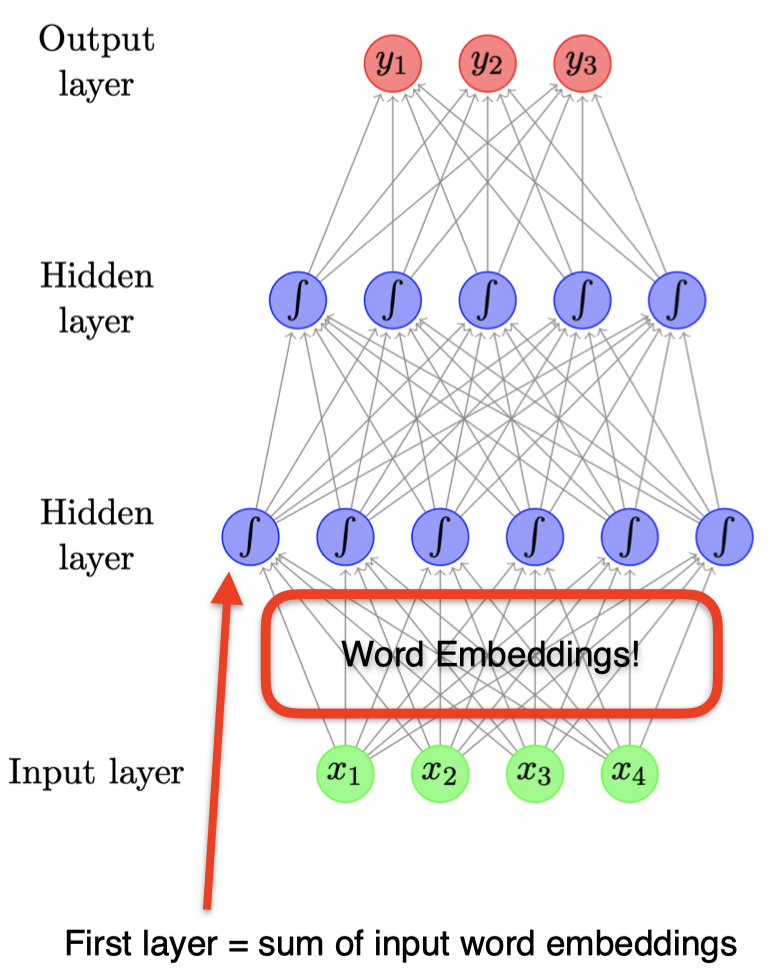### 3.5 语言模型架构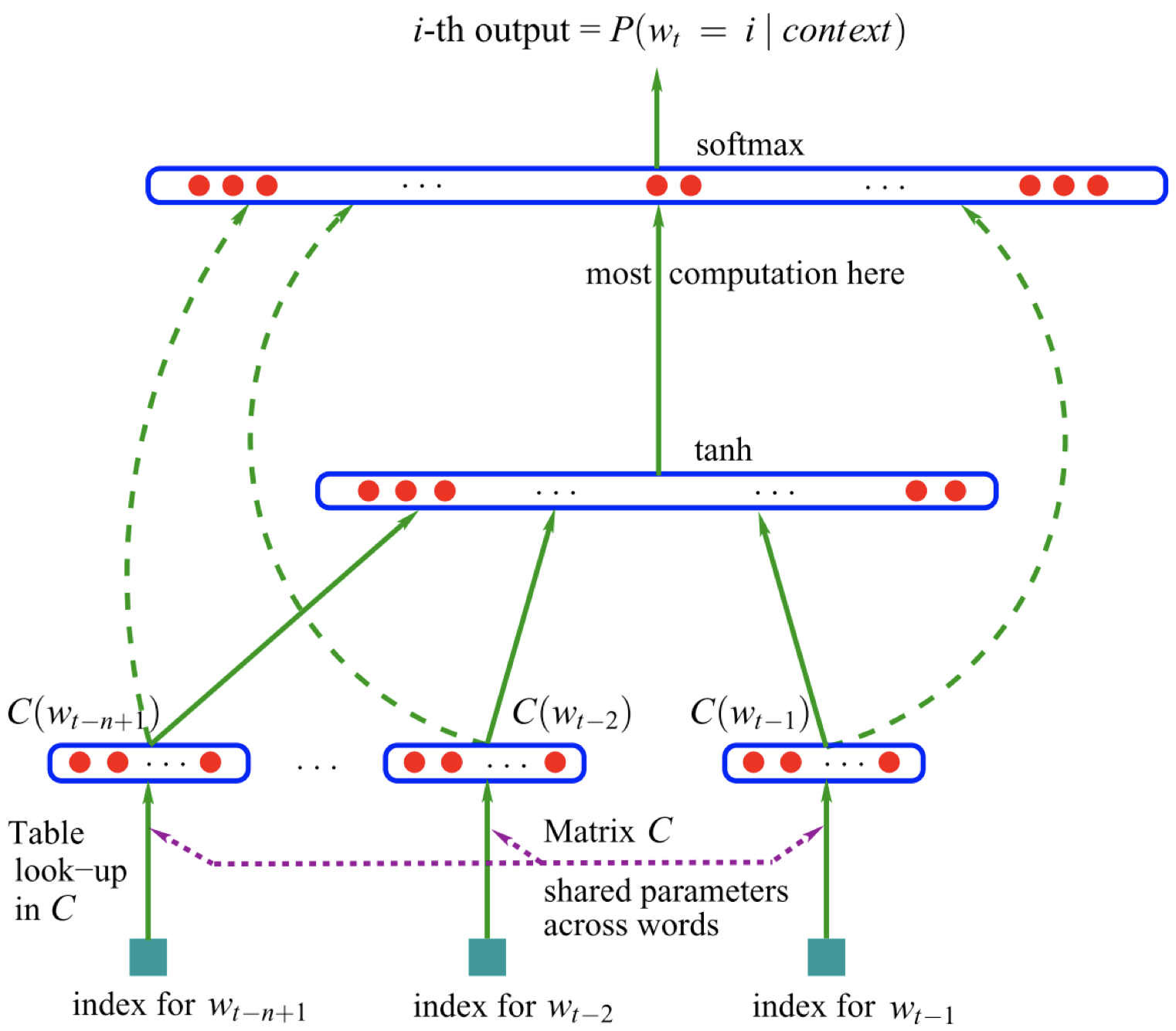$w_{t-n+1},\dots,w_{t-2},w_{t-1}$

$C(w_{t-n+1}),\dots,C(w_{t-2}),C(w_{t-1})$

### 3.6 例子

$P(w_i=“\textit{grass}”\mid w_{i-2}=“\textit{cow}”,w_{i-1}=“\textit{eats}”)$
• 查看单词 “$\textit{cow}$” 和 “$\textit{eats}$” 的词嵌入：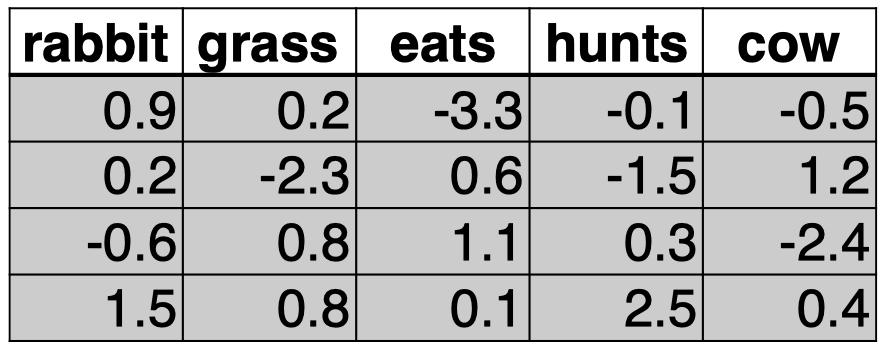可以看到，在这个例子中，我们的词汇表中大小为 $|V|=5$，词嵌入的维度 $d=4$。

• 将它们连接起来，输入网络中。

$\mathbf x=\mathbf v_{\textit{cow}}\oplus \mathbf v_{\textit{eats}}$ $\mathbf h_1=\tanh(W_1\mathbf x+\mathbf b_1)$ $\mathbf y=\text{softmax}(W_2\mathbf h_1)$

首先，我们将单词 “$\textit{cow}$” 和 “$\textit{eats}$” 的词嵌入 $\mathbf v_{\textit{cow}}$ 和 $\mathbf v_{\textit{eats}}$ 连接起来作为输入向量 $\mathbf x$。然后通过权重矩阵 $W_1$ 进行加权，并加上偏置向量 $\mathbf b_1$，将结果作为非线性函数 $\tanh$ 的输入，得到隐藏层的表示 $\mathbf h_1$。利用权重矩阵 $W_2$ 对 $\mathbf h_1$ 加权，并作为函数 $\text{softmax}$ 的输入，最终得到预测结果 $\mathbf y$。

• 输出：$\mathbf y$ 给出了词汇表中所有单词的概率分布。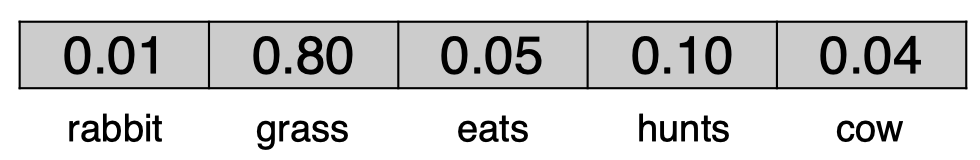$P(w_i=“\textit{grass}”\mid w_{i-2}=“\textit{cow}”,w_{i-1}=“\textit{eats}”)=0.8$

该前馈神经网络模型的大部分参数都在词嵌入（即所有单词的词嵌入表，维度为 $d\times |V|$）和输出嵌入（即 $W_2$，维度为 $|V|\times d$）中。

### 3.7 为什么要用神经网络构建语言模型

• N-grams 语言模型
• 训练成本低（只需要计算频数）
• 稀疏性问题，扩展到更大的上下文时存在问题
• 无法充分捕获单词特性（语法和语义上的相似度)，例如：$\textit{film}$ vs. $\textit{movie}$
• 神经网络语言模型（NNLMs）更具鲁棒性
• 强迫将单词用低维的嵌入表示
• 自动捕获单词特性，在计算句子概率时，使得估计更具鲁棒性
• 灵活性：只需稍加改变就可以适用于其他任务（例如：词性标注）

### 3.8 词性标注

• 词性标注（POS tagging）也可以被归为分类问题：

• 例如，给定当前单词 “$\textit{eats}$”，和它的前一个上下文单词 “$\textit{cow}$”，我们的目标是预测当前单词 “$\textit{eats}$” 的词性：

$P(t_i\mid w_{i-1}=\textit{“cow”},w_{i}=\textit{“eats”})$

对当前单词 “$\textit{eats}$” 的可能词性分类。

• 为什么不用一种更时髦的分类器呢？（神经网络）
• 神经网络语言模型架构可以直接适用于该任务。

### 3.9 前馈神经网络用于词性标注

• 最大熵马尔可夫模型（Maximum Entropy Markov Model, MEMM） 标注器：
• 输入：
• 最近的单词：$w_{i-2},w_{i-1},w_{i}$
• 最近的 tag：$t_{i-2},t_{i-1}$
• 输出：
• 当前单词 tag：$t_{i}$
• 神经网络 框架：
• 输入：
• 5 项：3 个词嵌入，2 个 tag 嵌入
• 输出：
• 1 项：长度为 $|T|$ 的向量（$T$ 为可能的 tags 集合），使用 $\text{softmax}$ 函数
• 训练过程：
• 最小化：$-\sum_{i}\log P(t_i\mid w_{i-2},w_{i-1},w_{i},t_{i-2},t_{i-1})$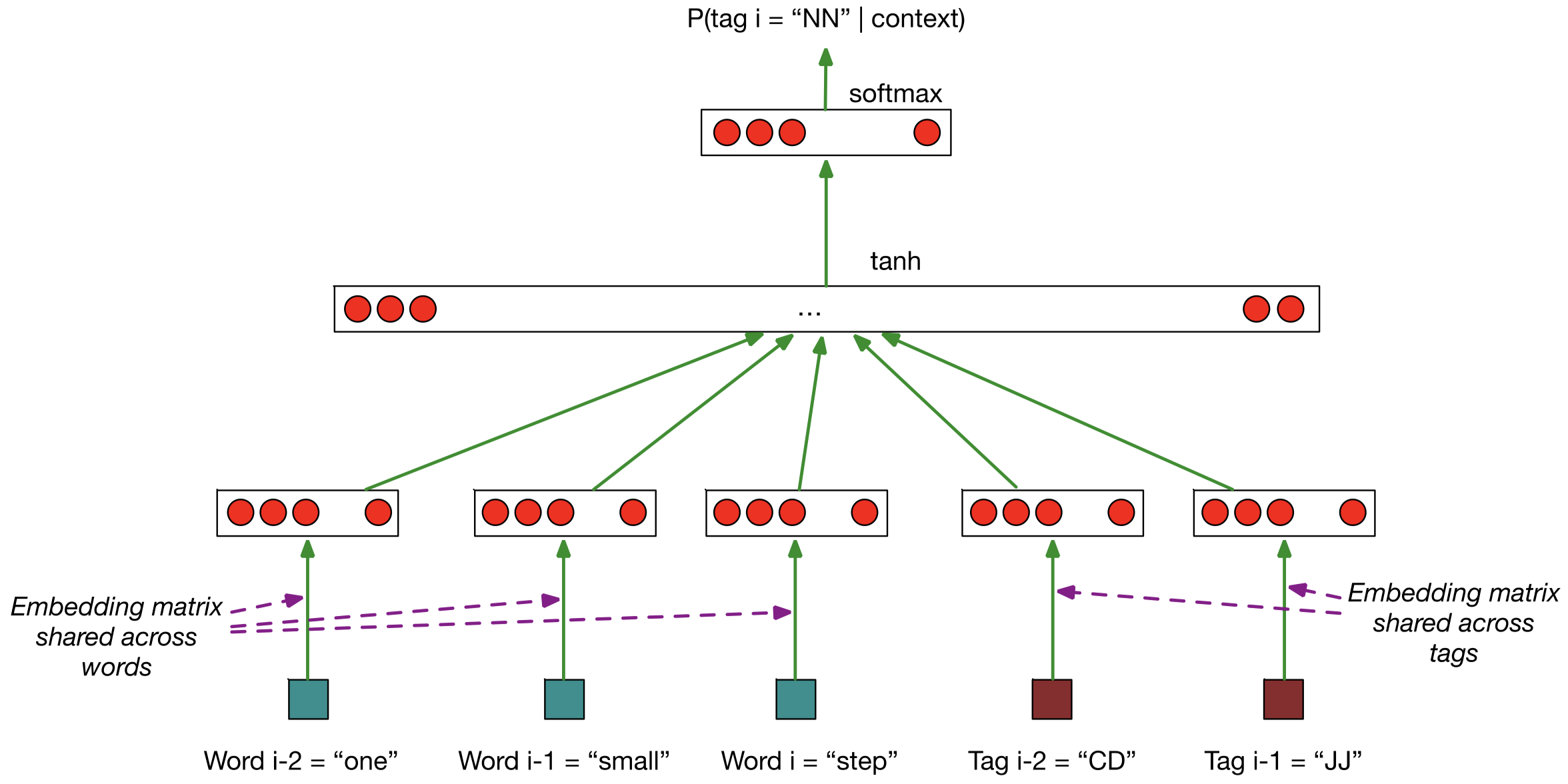这里，我们有 3 个单词：$w_{i-2}=\textit{“one”},w_{i-1}=\textit{“small”},w_{i}=\textit{“step”}$，我们将其编码为词嵌入，它们共用一个嵌入矩阵。同时，我们有上下文单词 $w_{i-2},w_{i-1}$ 对应的词性标签：$t_{i-2}=\text{“CD”},t_{i-1}=\text{“JJ”}$，同理，我们将其编码为 tag 嵌入，它们共用另一个嵌入矩阵。然后，我们将得到的 5 个嵌入向量（3 个词嵌入，2 个 tag 嵌入）连接得到一个长的向量，然后作为 $\tanh$ 函数的输入，然后将计算结果传入输出层。输出层的隐藏单元数量等于所有可能的 tags 组成的集合的大小。然后，我们将 $\text{softmax}$ 函数作用于该向量上，然后我们将得到所有可能的 tags 对应的概率向量，然后只需要取其中的最大值对应的 tag 作为当前单词 $w_{i}$ 的词性标签 $t_{i}$ 即可。

### 3.10 卷积神经网络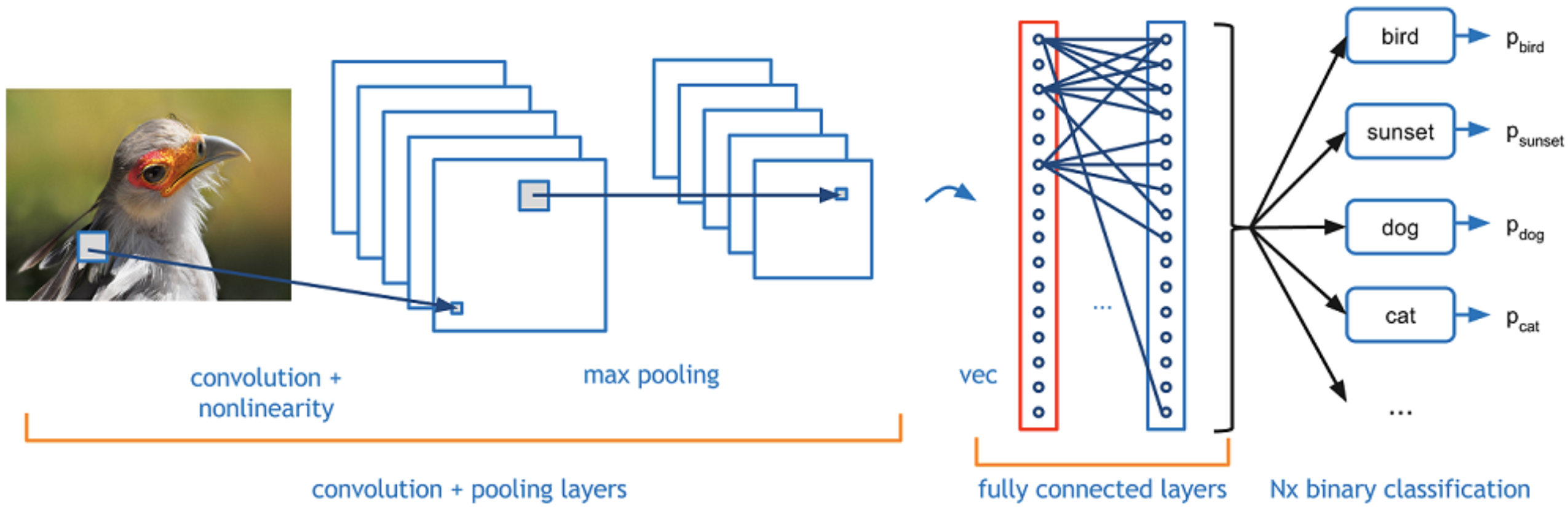• 通常用于 计算机视觉（Computer Vision, CV），但也可以用于 NLP 任务。
在 CV 任务中，输入为一张图片，它由很多 像素（pixel）组成。卷积神经网络的工作机制是：每次观察图片中的一小块区域，然后用 卷积核（convolution kernel）产生一个特征方阵。对于图片中的每一个小的区域，我们都可以进行这样的操作，这样我们得到了该图片的另一种表示。如果我们采用不同的卷积核，那么将得到不同的图片表示。为什么要使用不同的卷积核呢？因为这样我们可以从不同的潜在角度（例如：颜色、边缘锐度等）来捕获图片特征。之后，我们可以进行 最大池化（max pooling）操作，同样，我们观察图片表示中的一小块区域，取其中的最大值作为该区域的表示，对其他图片表示的每个小区域都进行同样的操作，这样我们得到一些新的特征表示。最后，将得到的特征表示喂给一个全连接层，然后我们将得到分类预测的结果。
• 识别有代表性特征的局部预测器。
这种方法之所以效果很好的原因在于，相比一次分析整张图片，我们采用了每次只分析其中的一小块区域，本质上看，我们每次只对这一小块区域进行预测，而最大池化操作使得模型可以只保留这块区域最明显的特征。
• 将这些局部预测器进行结合，生成一个固定大小的表示。

### 3.11 卷积神经网络用于 NLP 任务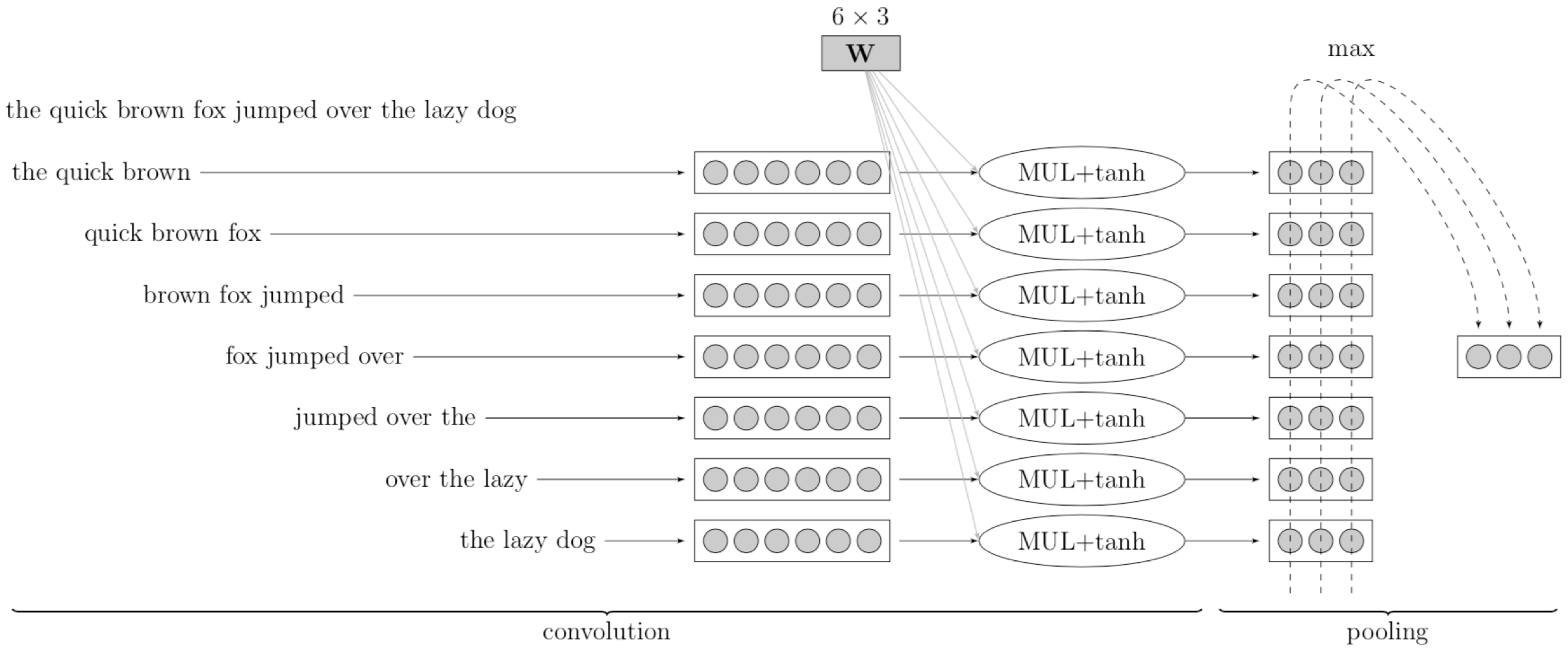• 在整个句子上的滑动窗口（例如：$3$ 个单词）
• $W$ 相当于卷积核（线性变换 + $\tanh$ 函数）
• 最大池化生成了一个固定大小的表示

## 4. 总结

• 神经网络
• 鲁棒性（例如：单词变体、拼写错误等）。
• 优秀的泛化能力。
• 灵活性 —— 基于不同任务定制不同的神经网络架构。
• 缺点
• 训练过程比传统机器学习方法要慢得多，但是可以通过 GPU 加速。
• 参数数量很多，主要受词汇表大小、嵌入、网络深度等因素影响。
• 对数据量需求很大（data hungry），在小型数据集上表现不是很好。
• 在大型语料库上的预训练模型（例如：BERT）可以缓解数据饥饿问题。

## 5. 扩展阅读

• Feed-forward network: G15, section 4
• Convolutional network: G15, section 9本作品采用知识共享署名-非商业性使用-相同方式共享 4.0 国际许可协议进行许可。 欢迎转载，并请注明来自：YEY 的博客 同时保持文章内容的完整和以上声明信息！